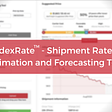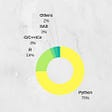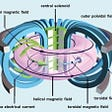# Analysing UK electricity prices (Part 2)

`import pandas as pdimport numpy as npsummary = {'mean': df.mean(), 'std': df.std(),           'min': df.min(), 'max': df.max()}summary = pd.DataFrame(summary)summary = summary.transpose()[['DA_H','DA_HH','Imbalance']]`
`import matplotlib.pyplot as pltcols = ['DA_H','DA_HH','Imbalance']data = [df[col] for col in cols]fig, ax = plt.subplots()labels=['{}\nmin={:3.1f}\nmax={:3.1f}'.format(col, df[col].min(), df[col].max()) for col in cols]ax.boxplot(data, whis=[5,95],            labels=labels,            showfliers=False,            medianprops={'color':'black'},            showmeans=True,           meanprops={'marker': 'x', 'markeredgecolor': 'black'})plt.show()`
`df['month'] = [d.month for d in df.date]data3 = []for i in range(1,13):    data3.append(df[df.month==i].DA_HH)    fig, ax = plt.subplots()ax.boxplot(data3, whis=[5,95],            showfliers=False,            medianprops={'color':'black'},            showmeans=True,            meanprops={'marker': 'x', 'markeredgecolor': 'black'})plt.show()`
`bands =  [(df.DA_HH.min(), 40), (40, 50), (50, 60),           (60,70), (70, 80), (80,90),           (90, df.DA_HH.max())]data2 = []labels2 = []for band in bands:    seg = df[(df.DA_HH>=band)&(df.DA_HH<band)].Imbalance    data2.append(seg)    labelstr = '{:3.0f}-{:3.0f}\nn={}\nmin={:3.0f}\nmax={:3.0f}'    labelstr = labelstr.format(band, band, len(seg),                                seg.min(), seg.max())    labels2.append(labelstr )fig, ax = plt.subplots()ax.boxplot(data2, whis=[5,95],            labels=labels2,            showfliers=False,            medianprops={'color':'black'},            showmeans=True,            meanprops={'marker': 'x', 'markeredgecolor': 'black'})plt.show()`

--

--

--

## More from Guy Lipman

Fascinated by what makes societies and markets work, especially in sustainable energy. http://guylipman.com. Views not necessarily reflect those of my employer.

Love podcasts or audiobooks? Learn on the go with our new app.

## Introducing dexRate V1.0## How to become a Machine Learning Engineer?## Stanford Entrepreneurs join One Concern Mission## Playing with the endowment effect in Python## Visualizations in Natural Language Processing## Using regression models to predict per capita and median household income in NYC## How to create your first Grafana Dashboard## House Price Predictions using Random Forest Regression## Guy Lipman

Fascinated by what makes societies and markets work, especially in sustainable energy. http://guylipman.com. Views not necessarily reflect those of my employer.

## Clean and Renewable? A Look Into a Future of Nuclear## What if Ice didn’t float on water?## Power, Unlimited Power! — A Primer on Fusion Energy